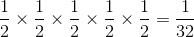# SSAT Middle Level Math : How to find the probability of an outcome

## Example Questions

← Previous 1

### Example Question #1 : Data Analysis And Probability

A pair of fair dice are rolled. What is the probability that the sum will be a multiple of 3?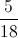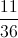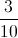Explanation:

There are four possible multiples of 3 that can come out: 3,6,9, and 12. There are 36 equally probable outcomes; the following will result in a multiple of 3: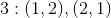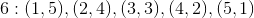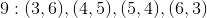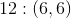These are 12 outcomes out of 36, making the probability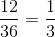### Example Question #2 : How To Find The Probability Of An Outcome

A pair of fair dice are rolled. What is the probability that the sum will be a multiple of 4?Explanation:

There are three possible multiples of 4 that can come out: 4, 8, and 12. There are 36 equally probable outcomes; the following will result in a multiple of 4: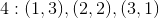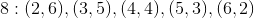These are 9 outcomes out of 36, making the probability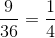### Example Question #1 : How To Find The Probability Of An Outcome

Some balls are placed in a hat - ten red, four blue, six yellow. What is the probability that a randomly drawn ball will not be blue?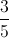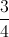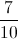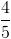Explanation:

There are 20 balls in the hat. All but four - that is, sixteen - are blue, so the probability of a draw resulting in a non-blue ball is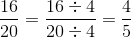### Example Question #1 : How To Find The Probability Of An Outcome

The red jacks are removed from a standard deck of fifty-two cards. What is the probability that a card randomly drawn from that modified deck will be black?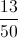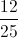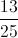Explanation:

The removal of two red jacks - and no black cards - results in there being fifty cards, twenty-six of them black. Therefore, the probability of a randomly drawn card being black is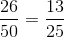.

### Example Question #1 : How To Find The Probability Of An Outcome

A standard deck of cards is modified by adding the red threes from another deck. What is the probability that a card randomly drawn from that modified deck will be a red card?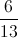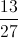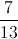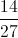Explanation:

The addition of two red threes from another deck results in the deck comprising fifty-four cards, twenty-eight of which are red. Therefore, the probability of a randomly drawn card being red is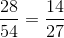### Example Question #1 : Probability

A standard deck of cards is modified by adding the red queens from another deck. What is the probability that a card randomly drawn from that modified deck will be a face card (jack, queen, king)?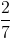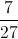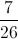Explanation:

There are four cards of each rank in a standard deck; since three ranks - jacks, queens, kings - are considered face cards, this makes twelve face cards out of the fifty-two. But two more face cards - two red queens - have been introduced, so now there are fourteen face cards out of fifty-four. This makes the probability of a randomly drawn card being a face card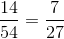### Example Question #2 : How To Find The Probability Of An Outcome

In a bag of marbles, there areblue marbles,red marbles, andgreen marbles. What is the probability of drawing two blue marbles in a row?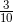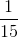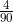3Explanation:

The probability of drawing a blue marble on the first try is, since there areblue marbles out of a total ofmarbles. The probability of drawing a second blue marble is, since now there areblue marbles remaining out of a total ofremaining marbles. The probability of drawing two blue marbles in a row is the product of the individual probabilities: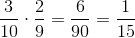.

### Example Question #1 : Data Analysis And Probability

Dave has a sock drawer with 8 blue and 10 black socks.

If Dave pulls out one black sock, what is the probability that the next sock he pulls out of the drawer is also black?

4 out of 9

10 out of 17

9 out of 17

1 out of 2

5 out of 9

9 out of 17

Explanation:

Since the first sock that Dave pulls out is black, there are 17 remaining socks in the drawer, 8 blue and 9 black. The probability that Dave will choose another black is sock is therefore 9 out of 17.

### Example Question #4 : Data Analysis And Probability

If Mark flips a coin and then rolls a die, what are the odds that the coin will be heads and that the die will land on a multiple of 3?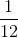Explanation:

If Mark flips a coin, the chance that it will land on heads is. On a die, there are 2 out of 6 numbers that are a multiple of 3 (3 and 6); therefore, there is achance that the dice will be a multiple of 3.

The probability that the coin will land on heads and that the dice will be a multiple of 3 is: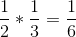### Example Question #5 : Probability

Lisa and Fred were flipping a quarter and recording whether it was heads or tails. What is the probability they flip a quarter and it lands on heads, heads, tails, heads, tails? (H,H,T,H,T)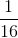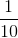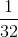There are two possibilities every time you flip a coin and only one outcome. Therefore the probability for flipping either heads or tails each time is. When you have multiple trials in a row you multiply the probabilities of each outcome by each other.